Next: Larmor Formula Up: Relativity and Electromagnetism Previous: Electromagnetic Energy Tensor

# Accelerated Charges

Let us calculate the electric and magnetic fields observed at position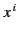and time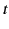due to a chargewhose retarded position and time areand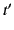, respectively. From now onis termed the field point and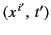is termed the source point. It is assumed that we are given the retarded position of the charge as a function of its retarded time: i.e.,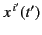. The retarded velocity and acceleration of the charge are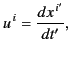(1889)

and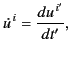(1890)

respectively. The radius vector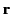is defined to extend from the retarded position of the charge to the field point, so that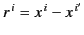. (Note that this is the opposite convention to that adopted in Sections 12.18 and 12.19). It follows that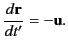(1891)

The field and the source point variables are connected by the retardation condition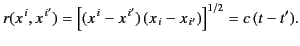(1892)

The potentials generated by the charge are given by the Liénard-Wiechert formulae,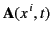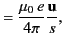(1893)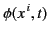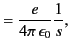(1894)

where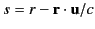is a function both of the field point and the source point variables. Recall that the Liénard-Wiechert potentials are valid for accelerating, as well as uniformly moving, charges.

The fields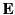and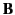are derived from the potentials in the usual manner: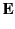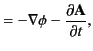(1895)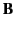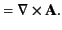(1896)

However, the components of the gradient operator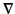are partial derivatives at constant time,, and not at constant time,. Partial differentiation with respect to thecompares the potentials at neighboring points at the same time, but these potential signals originate from the charge at different retarded times. Similarly, the partial derivative with respect toimplies constant, and, hence, refers to the comparison of the potentials at a given field point over an interval of time during which the retarded coordinates of the source have changed. Because we only know the time variation of the particle's retarded position with respect towe must transform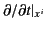and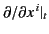to expressions involving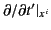and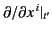.

Now, becauseis assumed to be given as a function of, we have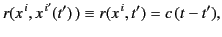(1897)

which is a functional relationship between,, and. Note that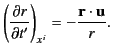(1898)

It follows that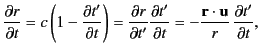(1899)

where all differentiation is at constant. Thus,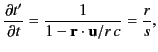(1900)

giving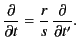(1901)

Similarly,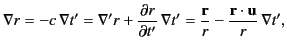(1902)

where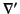denotes differentiation with respect toat constant. It follows that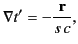(1903)

so that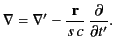(1904)

Equation (1897) yields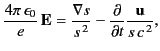(1905)

or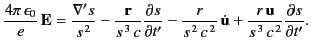(1906)

However,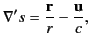(1907)

and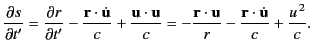(1908)

Thus,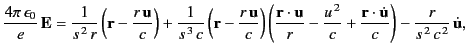(1909)

which reduces to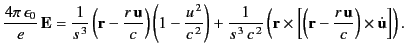(1910)

Similarly,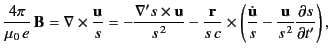(1911)

or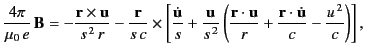(1912)

which reduces to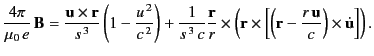(1913)

A comparison of Equations (1912) and (1915) yields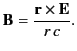(1914)

Thus, the magnetic field is always perpendicular toand the retarded radius vector. Note that all terms appearing in the previous formulae are retarded.

The electric field is composed of two separate parts. The first term in Equation (1912) varies asfor large distances from the charge. We can think of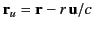as the virtual present radius vector: that is, the radius vector directed from the position the charge would occupy at timeif it had continued with uniform velocity from its retarded position to the field point. In terms of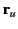, thefield is simply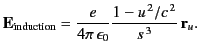(1915)

We can rewrite the expression (1835) for the electric field generated by a uniformly moving charge in the form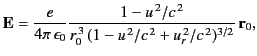(1916)

where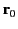is the radius vector directed from the present position of the charge at timeto the field point, and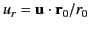. For the case of uniform motion, the relationship between the retarded radius vectorand the actual radius vectoris simply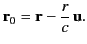(1917)

It is straightforward to demonstrate that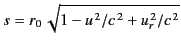(1918)

in this case. Thus, the electric field generated by a uniformly moving charge can be written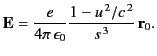(1919)

Because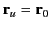for the case of a uniformly moving charge, it is clear that Equation (1917) is equivalent to the electric field generated by a uniformly moving charge located at the position the charge would occupy if it had continued with uniform velocity from its retarded position.

The second term in Equation (1912),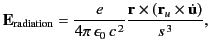(1920)

is of order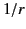, and, therefore, represents a radiation field. Similar considerations hold for the two terms of Equation (1915).Next: Larmor Formula Up: Relativity and Electromagnetism Previous: Electromagnetic Energy Tensor
Richard Fitzpatrick 2014-06-27